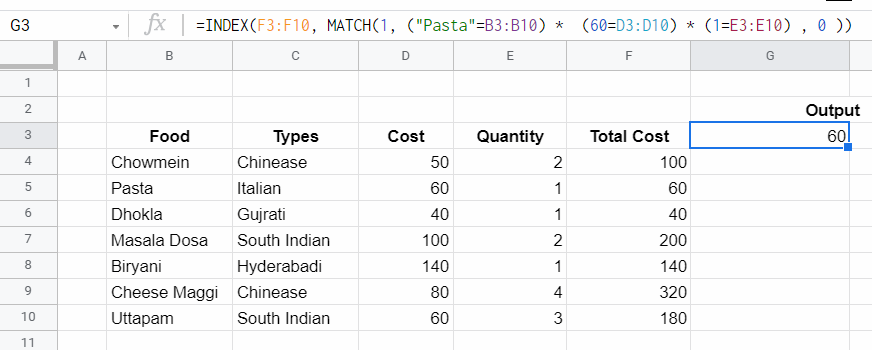Open In App

# INDEX and MATCH Function in Excel

INDEX-MATCH has become a more popular tool for Excel as it solves the limitation of the VLOOKUP function, and it is easier to use. INDEX-MATCH function in Excel has a number of advantages over the VLOOKUP function:

1. INDEX and MATCH are more flexible and faster than Vlookup
2. It is possible to execute horizontal lookup, vertical lookup, 2-way lookup, left lookup, case-sensitive lookup, and even lookups based on multiple criteria.
3. In sorted Data, INDEX-MATCH is 30% faster than VLOOKUP. This means that in a larger dataset 30% faster makes more sense.

Let’s begin with the detailed concepts of each INDEX and MATCH.

### INDEX Function

The INDEX function in Excel is very powerful at the same time a flexible tool that retrieves the value at a given location in a range. In another word, It returns the content of a cell, specified by row and column offset.

Syntax:

`=INDEX(reference, [row], [column])`

Parameters:

• reference: The array of cells to be offset into. It can be a single range or an entire dataset in a table of data.
• row [optional]: The number of offset rows. It means if we choose a table reference range as “A1:A5” then the Cell/content that we want to extract is at how much vertical distance. Here, for A1 row will be 1, for A2 row = 2, and so on. If we give row = 4 then it will extract A4. As row is optional so if we don’t specify any row number then it extracts entire rows in the reference range. That is A1 to A5 in this case.
• column [optional]: The number of offset columns. It means if we choose a table reference range as “A1:B5” then the Cell/content we want to extract is at how much horizontal distance. Here, for A1 row will be 1  and column will be 1, for B1 row will be 1 but the column will be 2 similarly for A2 row = 2 column = 1, for B2 row = 2 column = 2 and so on. If we give row = 5 and column 2 then it will extract B5. As the column is optional so if we don’t specify any row no. then it will extract the entire column in the reference range. For example, if we give row = 2 and column as empty then it will extract (A2:B2). If we don’t specify Row and column both then it will extract the entire reference table that is (A1:B5).

Reference Table: The following table will be used as a reference table for all the examples of the INDEX function. First Cell is at B3 (“FOOD”) and the Last Diagonal Cell is at F10 (“180”).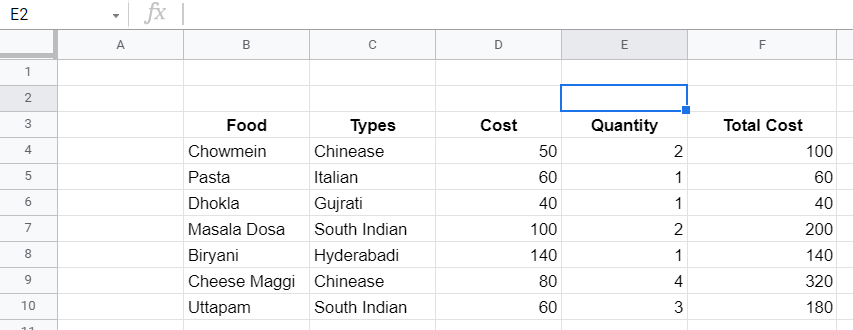Examples: Below are some examples of Index functions.

Case 1: No Rows and Columns are mentioned.

Input Command: =INDEX(B3:C10)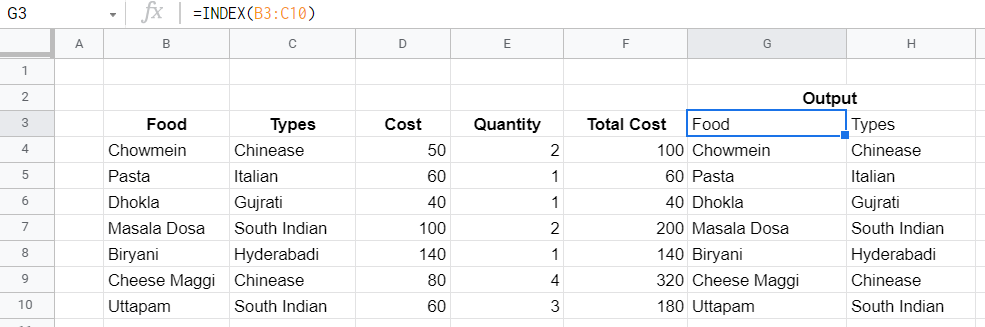Case 2: Only Rows are Mentioned.

Input Command: =INDEX(B3:C10,2)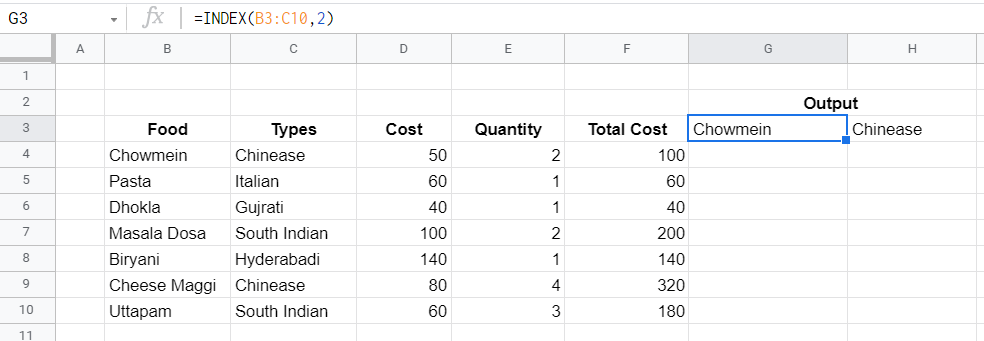Case 3: Both Rows And Columns are mentioned.

Input Command: =INDEX(B3:D10,4,2)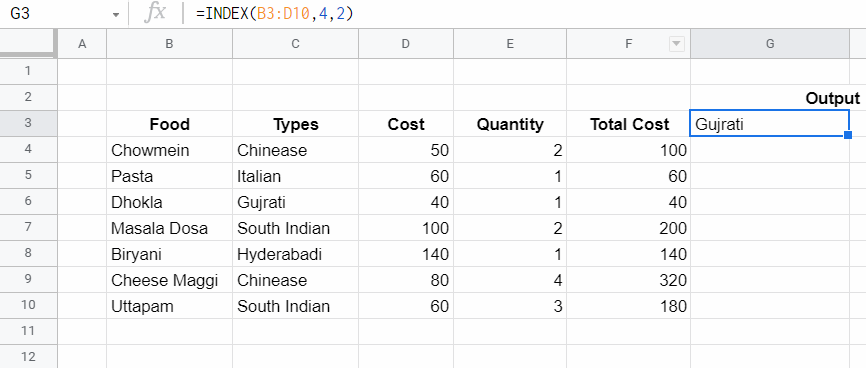Case 4: Only Columns are mentioned.

Input Command: =INDEX(B3 : D10 , , 2)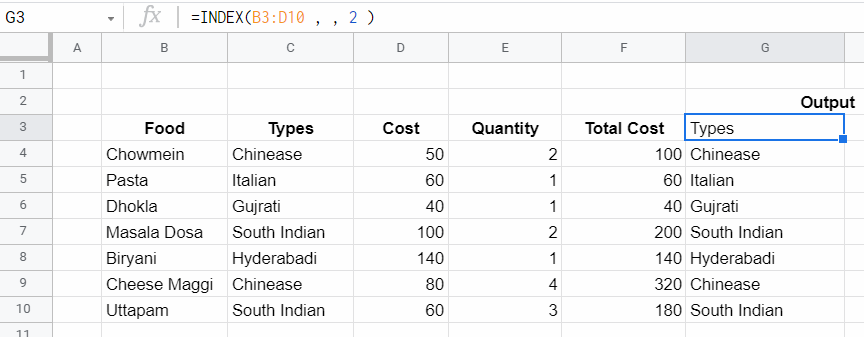Problem With INDEX Function: The problem with the INDEX function is that there is a need to specify rows and columns for the data that we are looking for. Let’s assume we are dealing with a machine learning dataset of 10000 rows and columns then it will be very difficult to search and extract the data that we are looking for. Here comes the concept of Match Function, which will identify rows and columns based on some condition.

### MATCH Function

It retrieves the position of an item/value in a range. It is a less refined version of a VLOOKUP or HLOOKUP that only returns the location information and not the actual data.  MATCH is not case-sensitive and does not care whether the range is Horizontal or Vertical.

Syntax:

`=MATCH(search_key, range, [search_type])`

Parameters:

• search_key: The value to search for. For example, 42, “Cats”, or I24.
• range: The one-dimensional array to be searched. It Can Either be a single row or a single column.eg->A1:A10 , A2:D2 etc.
• search_type [optional]: The search method. = 1 (default) finds the largest value less than or equal to search_key when the range is sorted in ascending order.
• = 0 finds the exact value when the range is unsorted.
• = -1 finds the smallest value greater than or equal to search_key when the range is sorted in descending order.

Row number or Column number can be found using the match function and can use it inside the index function so if there is any detail about an item, then all information can be extracted about the item by finding the row/column of the item using match then nesting it into index function.

Reference Table: The following table will be used as a reference table for all the examples of the MATCH function. First Cell is at B3 (“FOOD”) and the Last Diagonal Cell is At F10 (“180”)Examples: Below are some examples of the MATCH function-

Case 1: Search Type 0, It means Exact Match.

Input Command: =MATCH(“South Indian”,C3:C10,0)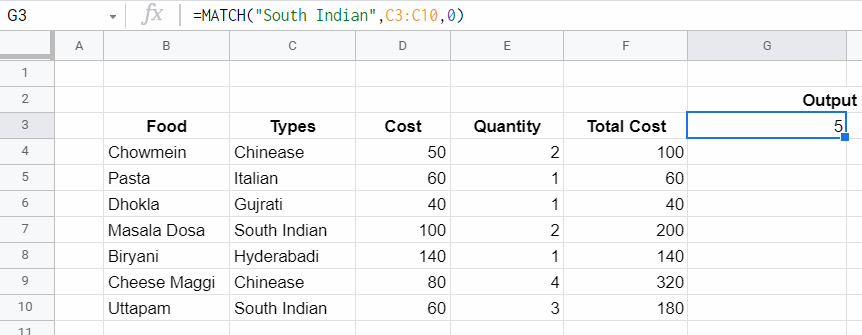Case 2: Search Type 1 (Default).

Input Command: =MATCH(“South Indian”,C3:C10)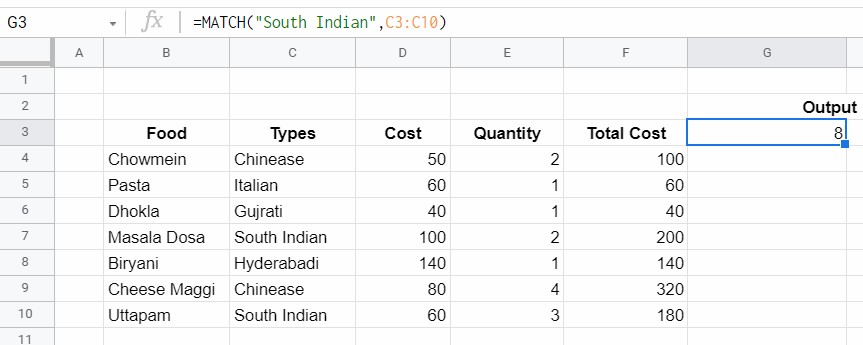Case 3: Search Type -1.

Input Command: =MATCH(“South Indian”,C3:C10,-1)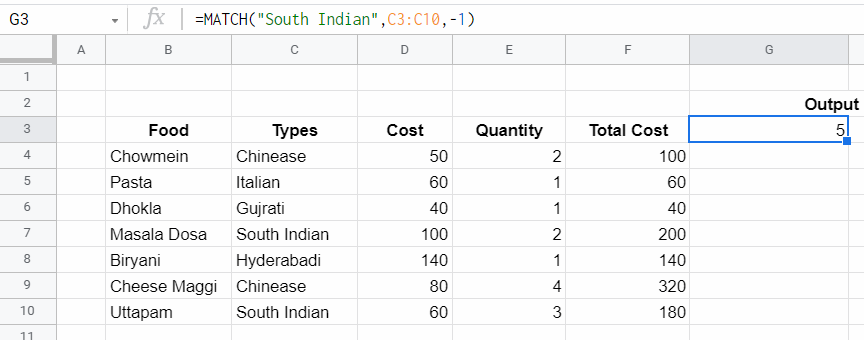### INDEX-MATCH Together

In the previous examples, the static values of rows and columns were provided in the INDEX function Let’s assume there is no prior knowledge about the rows and column position then rows and columns position can be provided using the MATCH function. This Is a dynamic way to search and extract value.

Syntax:

```=INDEX(Reference Table , [Match(SearchKey,Range,Type)/StaticRowPosition],
[Match(SearchKey,Range,Type)/StaticColumnPosition])```

Reference Table: The following reference table will be used. First Cell is at B3 (“FOOD”) and the Last Diagonal Cell is At F10 (“180”)Example: Let’s say the task is to find the cost of Masala Dosa. It is known that column 3 represents the cost of items, but the row position of Masala Dosa is not known. The problem can be divided into two steps-

Step 1: Find the position of Masala Dosa by using the formula:

`=MATCH("Masala Dosa",B3:B10,0) `

Here B3:B10 represents Column “Food” and 0 means Exact Match. It will return the row number of Masala Dosa.

Step 2: Find the cost of Masala Dosa. Use the INDEX Function to find the cost of Masala Dosa. By substituting the above MATCH function query inside the INDEX function at the place where the exact position of Masala Dosa is required, and the column number of cost is 3 which is already known.

`=INDEX(B3:F10, MATCH("Masala Dosa", B3:B10 , 0) ,3)`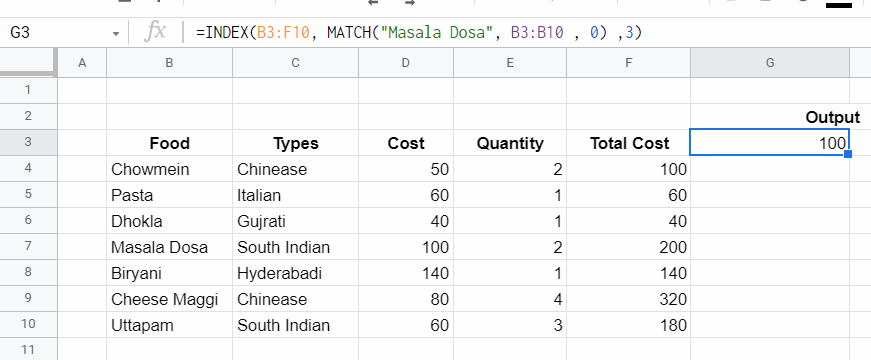### Two Ways Lookup With INDEX-MATCH Together

In the previous example, the column position Of the “Cost” attribute was hardcoded. So, It was not fully dynamic.

Case 1: Let’s assume there is no knowledge about the column number of Cost also, then it can be obtained using the formula:

`=MATCH("Cost",B3:F3,0)`

Case 2: When row, as well as column value, are provided via MATCH function (without giving static value) then it is called Two-Way Lookup. It can be achieved using the formula:

`=INDEX(B3:F10, MATCH("Masala Dosa",B3:B10, 0) , MATCH("Cost" ,B3:F3 ,0))`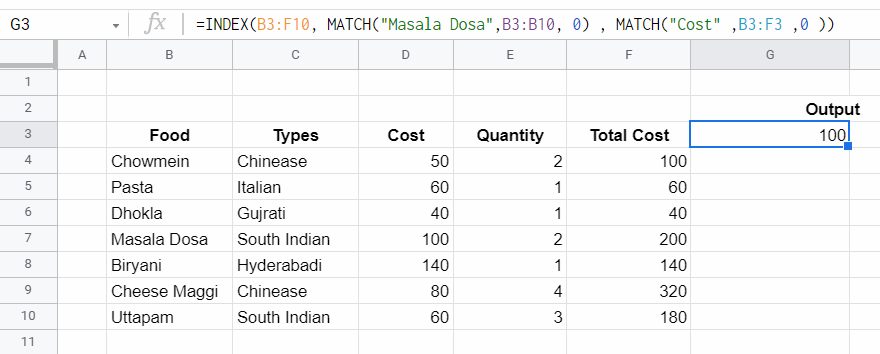### Left Lookup

One of the key advantages of INDEX and MATCH over the VLOOKUP function is the ability to perform a “left lookup”. It means it is possible to extract the row position of an item from using any attribute at right and the value of another attribute in left can be extracted.

For Example, Let’s say buy food whose cost should be 140 Rs. Indirectly we are saying buy “Biryani”. In this example, the cost Rs 140/- is known, there is a need to extract the “Food”. Since the Cost column is placed to the right of the Food column. If VLOOKUP is applied it will not be able to search the left side of the Cost column. That is why using VLOOKUP it is not possible to get Food Name.

To overcome this disadvantage INDEX-MATCH function Left lookup can be used.
Step 1: First extract row position of Cost 140 Rs using the formula:

`=MATCH(140, D3:D10,0)`

Here D3: D10 represents the Cost column where the search for the Cost 140 Rs row number is being done.

Step 2: After getting the row number, the next step is to use the INDEX Function to extract Food Name using the formula:

`=INDEX(B3:B10, MATCH(140, D3:D10,0)) `

Here B3:B10 represents Food Column and 140 is the Cost of the food item.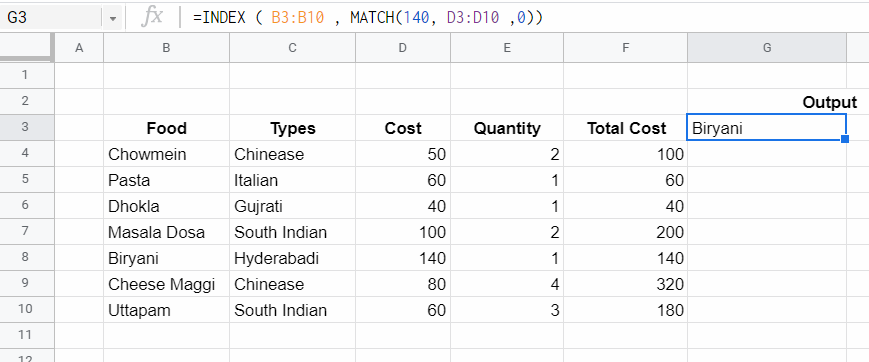### Case Sensitive Lookup

By itself, the MATCH function is not case-sensitive. This means if there is a Food Name “DHOKLA” and the MATCH function is used with the following search word:

1. “Dhokla”
2. “dhokla”
3. “DhOkLA”

All will return the row position of DHOKLA. However, the EXACT function can be used with INDEX and MATCH to perform a lookup that respects upper and lower case.

Exact Function: The Excel EXACT function compares two text strings, taking into account upper and lower case characters, and returns TRUE if they are the same, and FALSE if not. EXACT is case-sensitive.

Examples:

1. EXACT(“DHOKLA”,”DHOKLA”): This will return True.
2. EXACT(“DHOKLA”,”Dhokla”): This will return False.
3. EXACT(“DHOKLA”,”dhokla”): This will return False.
4. EXACT(“DHOKLA”,”DhOkLA”): This will return False.

Example: Let say the task is to search for the Type Of Food “Dhokla” but in Case-Sensitive Way. This can be done using the formula-

`=INDEX(C3:C10, MATCH(TRUE , EXACT("Dhokla", B3:B10) ,0))`

Here the EXACT function will return True if the value in Column B3:B10 matches with “Dhokla” with the same case, else it will return False. Now MATCH function will apply in Column B3:B10 and search for a row with the Exact value TRUE. After that INDEX Function will retrieve the value of Column C3:C10 (Food Type Column) at the row returned by the MATCH function.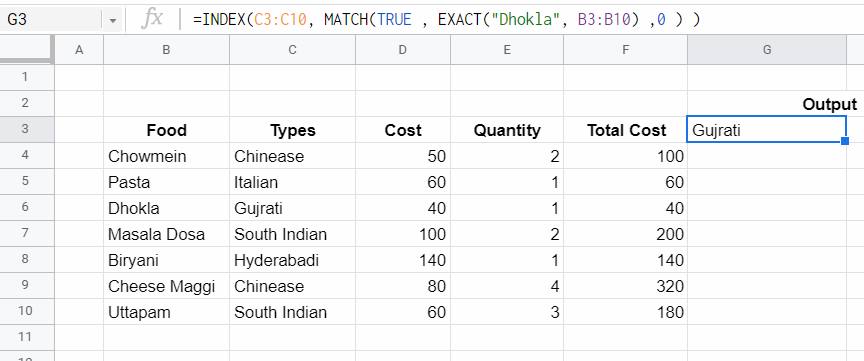### Multiple Criteria Lookup

One of the trickiest problems in Excel is a lookup based on multiple criteria. In other words, a lookup that matches on more than one column at the same time. In the example below, the INDEX and MATCH function and boolean logic are used to match on 3 columns-

1. Food.
2. Cost.
3. Quantity.

To extract total cost.

Example: Let’s say the task is to calculate the total cost of Pasta where

1. Food: Pasta.
2. Cost: 60.
3. Quantity: 1.

So in this example, there are three criteria to perform a Match. Below are the steps for the search based on multiple criteria-

Step 1: First match Food Column (B3:B10) with Pasta using the formula:

`"PASTA" = B3:B10 `

This will convert B3:B10 (Food Column) values as Boolean. That Is True where Food is Pasta else False.

Step 2: After that, match Cost criteria in the following manner:

`60 = D3:D10`

This will replace D3:D10 (Cost Column) values as Boolean. That is True where Cost=60 else False.

Step 3: Next step is to match the third criteria that are Quantity = 1 in the following manner:

`1 = E3:E10`

This will replace E3:E10 Column (Quantity Column) as True where Quantity = 1 else it will be False.

Step 4: Multiply the result of the first, second, and third criteria. This will be the intersection of all conditions and convert Boolean True / False as 1/0.

Step 5: Now the result will be a Column with 0 And 1. Here use the MATCH Function to find the row number of columns that contain 1. Because if a column is having the value 1, then it means it satisfies all three criteria.

Step 6: After getting the row number use the INDEX function to get the total cost of that row.

`=INDEX(F3:F10, MATCH(1, ("Pasta"=B3:B10) *  (60=D3:D10) * (1=E3:E10) , 0 ))`

Here F3:F10 represents the Total Cost Column.